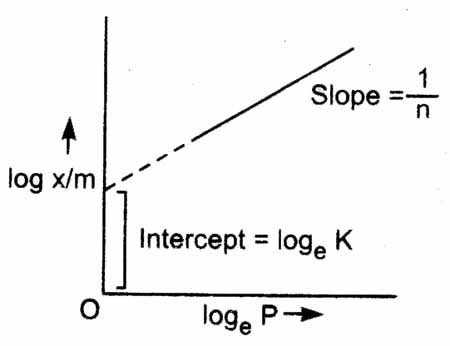#### For adsorption of a gas on a solid, the plot of logVs log p is linear with slope equal to Option 1) K Option 2) log K Option 3) n Option 4)As we learned inc oncept

Logarithmic graph of Freundlich isotherm -- wherein

The slope of the straight line gives the value of 1/n. The intercept on y-axis gives the value of log K.

Isotherm,Plotting the above equation in graph, we get

The slope gives the value of 1/x

Option 1)

K

Incorrect option

Option 2)

log K

Incorrect option

Option 3)

n

Incorrect option

Option 4)Correct option